The variation of anode current in a triode corresponding to a change in grid potential at three different values of the plate potential is shown in the diagram. The mutual conductance of the triode is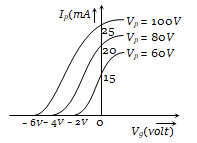(a) 2.5 m mho      (b) 5.0 m mho
(c) 7.5 m mho      (d) 10.0 m mho

Concept Questions :-

Transistor
High Yielding Test Series + Question Bank - NEET 2020

Difficulty Level:

A silicon speciman is made into a P-type semi-conductor by dopping, on an average, one Indium atom per $5×{10}^{7}$ silicon atoms. If the number density of atoms in the silicon specimen is  then the number of acceptor atoms in silicon per cubic centimetre will be

(a)                    (b)
(c)                    (d)

Concept Questions :-

Types of semiconductors
High Yielding Test Series + Question Bank - NEET 2020

Difficulty Level:

The probability of electrons to be found in the conduction band of an intrinsic semiconductor at a finite temperature

(a) Decreases exponentially with increasing band gap
(b) Increases exponentially with increasing band gap
(c) Decreases with increasing temperature
(d) Is independent of the temperature and the band gap

Concept Questions :-

Energy band theory
High Yielding Test Series + Question Bank - NEET 2020

Difficulty Level:

A 2V battery is connected across the points A and B as shown in the figure given below. Assuming that the resistance of each diode is zero in forward bias and infinity in reverse bias, the current supplied by the battery when its positive terminal is connected to A is(a) 0.2 A
(b) 0.4 A
(c) Zero
(d) 0.1 A

Concept Questions :-

PN junction
High Yielding Test Series + Question Bank - NEET 2020

Difficulty Level:

In the circuit, if the forward voltage drop for the diode is 0.5V, the current will be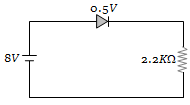(a) 3.4 mA
(b) 2 mA
(c) 2.5 mA
(d) 3 mA

Concept Questions :-

PN junction
High Yielding Test Series + Question Bank - NEET 2020

Difficulty Level:

Current in the circuit will be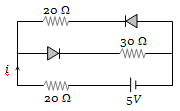(a) $\frac{5}{40}\mathrm{A}$
(b) $\frac{5}{50}\mathrm{A}$
(c) $\frac{5}{10}\mathrm{A}$
(d) $\frac{5}{20}\mathrm{A}$

Concept Questions :-

Applications of PN junction
High Yielding Test Series + Question Bank - NEET 2020

Difficulty Level:

The diode used in the circuit shown in the figure has a constant voltage drop of 0.5 V at all currents and a maximum power rating of 100 milliwatts. What should be the value of the resistor R, connected in series with the diode for obtaining maximum current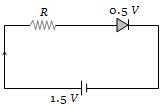(a) 1.5 $\mathrm{\Omega }$
(b) 5 $\mathrm{\Omega }$
(c) 6.67 $\mathrm{\Omega }$
(d) 200 $\mathrm{\Omega }$

Concept Questions :-

Applications of PN junction
High Yielding Test Series + Question Bank - NEET 2020

Difficulty Level:

In the following common emitter configuration an NPN transistor with current gain $\mathrm{\beta }$ = 100 is used. The output voltage of the amplifier will be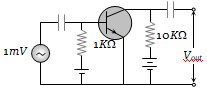(a) 10 mV
(b) 0.1 V
(c) 1.0 V
(d) 10 V

Concept Questions :-

Transistor
High Yielding Test Series + Question Bank - NEET 2020

Difficulty Level:

A sinusoidal voltage of peak value 200 volt is connected to a diode and resistor R in the circuit shown so that half wave rectification occurs. If the forward resistance of the diode is negligible compared to R the rms voltage (in volt) across R is approximately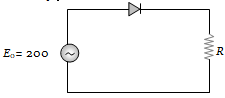(a) 200                            (b) 100
(c) $\frac{200}{\sqrt{2}}$                          (d) 280

Concept Questions :-

PN junction
High Yielding Test Series + Question Bank - NEET 2020

Difficulty Level:

The junction diode in the following circuit requires a minimum current of 1 mA to be above the knee point (0.7 V) of its I-V characteristic curve. The voltage across the diode is independent of current above the knee point. If ${\mathrm{V}}_{\mathrm{B}}$ = 5 V, then the maximum value of R so that the voltage is above the knee point, will be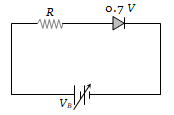(a) 4.3 k$\mathrm{\Omega }$                 (b) 860 k$\mathrm{\Omega }$

(c) 4.3 $\mathrm{\Omega }$                   (d) 860 $\mathrm{\Omega }$

Concept Questions :-

PN junction